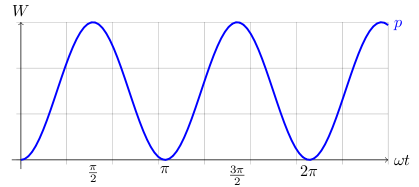# RMS (Current and Voltage)

Consider the power versus time graph of an AC signal:The average power, Pavg, for one cycle is the area under the graph divided by 2π. So if 0≤θ≤2π, p is the instantaneous power, i is the instantaneous current, and I0 is the peak current, then

```Pavg = 1/2π×∫02πpidθ
= 1/2π×∫02πi2R dθ
= R/2π×∫02πi2dθ
= R/2π×∫02πI02sin2θ dθ
= I02R/2π×∫02πsin2θ dθ
= I02R/2π×π
= I02R/2
```

In the case of sinusoidal current, the Root Mean Square (RMS) of an AC source is the DC source that gives the same average power.

```IRMS = I0/√2
```

and if the peak voltage of the AC signal is V0, then

```VRMS = V0/√2
```

which gives us

```Pavg = IRMS2R
Pavg = IRMSVRMS
```

## References

Fischer-Cripps. A.C., The Electronics Companion. Institute of Physics, 2005.